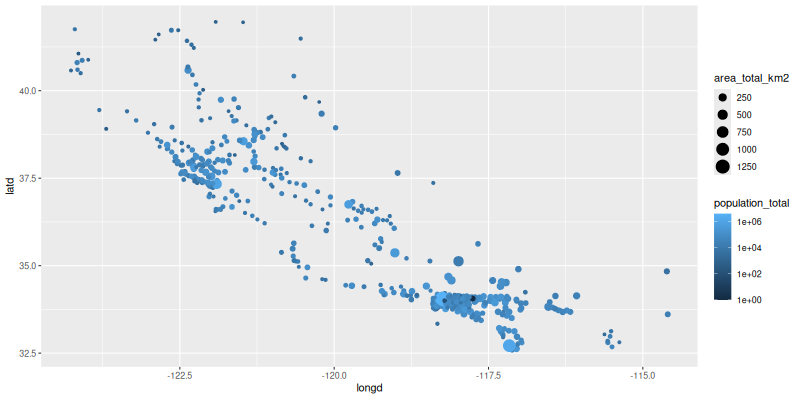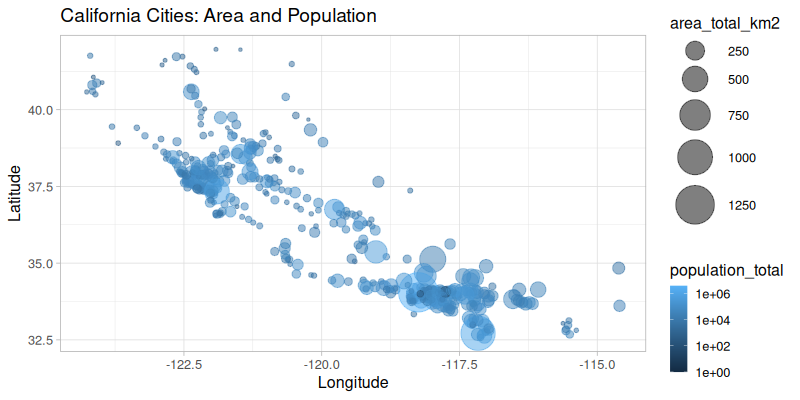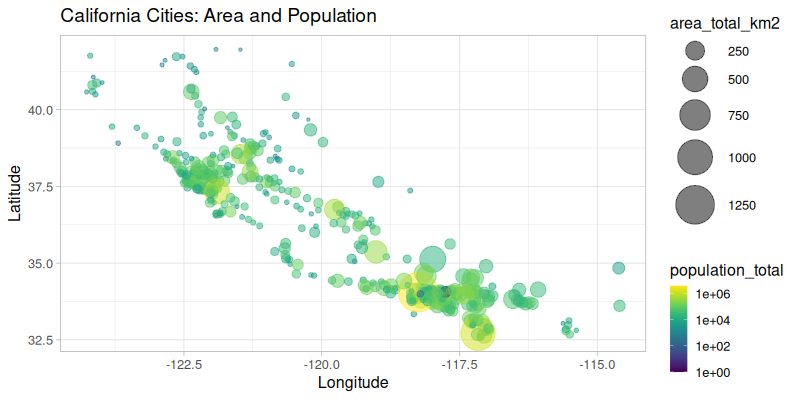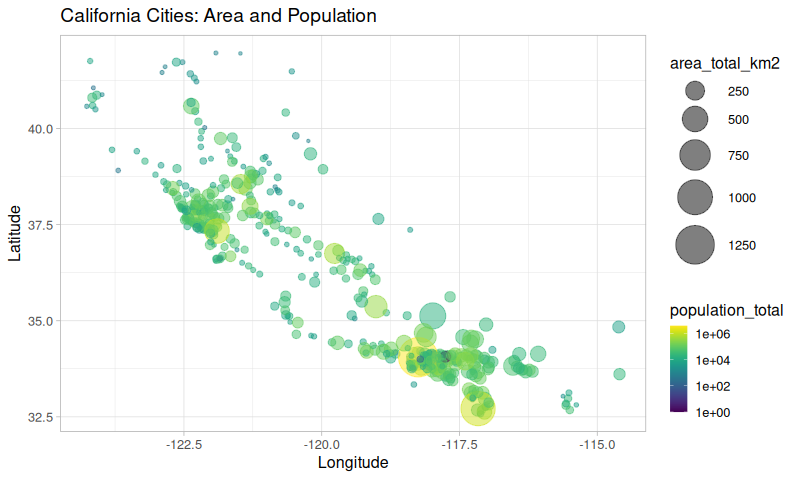# Using rpy2 in notebooks¶

rpy2 is designed to play well with notebooks. While the target notebook is jupyter, it is used in other notebook systems (some of them are based on Jupyter, or are managed Jupyter notebook systems).

rpy2 is working, and is sometimes available by default, with Google’s Colab, Databricks notebooks, AWS SageMaker, Azure Notebooks, or Google Cloud AI Platform Notebooks.

This section shows how rpy2 can be used to add everything R can offer to Python notebooks.

Note

This section is available as a jupyter notebook jupyter.ipynb (HTML render: jupyter.html)

```from functools import partial
from rpy2.ipython import html
html.html_rdataframe=partial(html.html_rdataframe, table_class="docutils")
```

## Data Import¶

We choose to use an external dataset to demonstrate how R’s own data import features can be used.

```from rpy2.robjects.packages import importr
utils = importr('utils')

'master/notebooks_v1/data/california_cities.csv')
```

The objects returned by R’s own `read.csv()` function (note that the R function in the R package `utils` is called `read.csv()` while the Python function is called `read_csv()` - `rpy2` converts R symbols with dots to underscores for Python).

`rpy2` provides customization to display R objects such as data frames in HTML in a notebook. That customization is enabled as follows:

```import rpy2.ipython.html
rpy2.ipython.html.init_printing()
```
```dataf
```
DataFrame with 482 rows and 14 columns:
X city latd longd ... area_water_km2 area_water_percent
0 1 0 Adelanto 34.57611111111112 -117.43277777777779 ... 0.046 0.03
1 2 1 AgouraHills 34.15333333333333 -118.76166666666667 ... 0.076 0.37
2 3 2 Alameda 37.75611111111111 -122.27444444444444 ... 31.983 53.79
3 4 3 Albany 37.886944444444445 -122.29777777777778 ... 9.524 67.28
4 5 4 Alhambra 34.081944444444446 -118.135 ... 0.003 0.01
5 6 5 AlisoViejo 33.575 -117.72555555555556 ... 0.0 0.0
6 7 6 Alturas 41.48722222222222 -120.5425 ... 0.036000000000000004 0.57
7 8 7 AmadorCity 38.419444444444444 -120.82416666666666 ... 0.0 0.0
... ... ... ... ... ... ... ... ...
480 481 480 Yucaipa 34.030277777777776 -117.04861111111111 ... 0.013000000000000001 0.02
481 482 481 YuccaValley 34.13333333333333 -116.41666666666667 ... 0.0 0.0
```dataf.colnames
```
StrVector with 14 elements:
 X city latd longd elevation_m elevation_ft population_total area_total_sq_mi ... area_water_km2 area_water_percent
```stats = importr('stats')
base = importr('base')
fit = stats.lm('elevation_m ~ latd + longd', data=dataf)
fit
```
ListVector with 13 elements:
 0 coefficients (Intercep... 1 residuals ... 2 effects ... 3 rank  3 4 fitted.values ... 5 assign  0 1 2 6 qr \$qr ... 7 df.residual  431 ... ... ... 11 terms elevation... 12 model ...

## Graphics¶

R has arguably some the best static visualizations, often looking more polished than other visualization systems and this without the need to spend much effort on them.

### Using `ggplot2`¶

Among R visulization pacakges, `ggplot2` has emerged as something Python users wished so much they had that various projects to try port it to Python are regularly started.

However, the best way to have `ggplot2` might be to use `ggplot2` from Python.

```import rpy2.robjects.lib.ggplot2 as gp
```
```/opt/hostedtoolcache/Python/3.9.17/x64/lib/python3.9/site-packages/rpy2/robjects/lib/ggplot2.py:70: UserWarning: This was designed againt ggplot2 versions starting with 3.3. but you have 3.4.2
warnings.warn(
```

R lets is function parameters be unevaluated language objects, which is fairly different from Python’s immediate evaluation. `rpy2` has a utility code to create such R language objects from Python strings. It can then become very easy to mix Python and R, with R like a domain-specific language used from Python.

```from rpy2.robjects import rl
```

Calling `ggplot2` looks pretty much like it would in R, which allows one to use the all available documentation and examples available for the R package. Remember that this is not a reimplementation of ggplot2 with inevitable differences and delay for having the latest changes: the R package itself is generating the figures.

```p = (gp.ggplot(dataf) +
gp.aes(x=rl('longd'),
y=rl('latd'),
color=rl('population_total'),
size=rl('area_total_km2')) +
gp.geom_point() +
gp.scale_color_continuous(trans='log10'))
```

Plotting the resulting R/ggplot2 object into the output cell of a notebook, is just function call away.

```from rpy2.ipython.ggplot import image_png
image_png(p)
```
```R[write to console]: In addition:
R[write to console]: Warning messages:

R[write to console]: 1:
R[write to console]: In (function (package, help, pos = 2, lib.loc = NULL, character.only = FALSE,  :
R[write to console]:

R[write to console]:  library ‘/home/runner/work/_temp/Library’ contains no packages

R[write to console]: 2:
R[write to console]: In (function (package, help, pos = 2, lib.loc = NULL, character.only = FALSE,  :
R[write to console]:

R[write to console]:  library ‘/home/runner/work/_temp/Library’ contains no packages

R[write to console]: 3:
R[write to console]: In (function (package, help, pos = 2, lib.loc = NULL, character.only = FALSE,  :
R[write to console]:

R[write to console]:  library ‘/home/runner/work/_temp/Library’ contains no packages

R[write to console]: 4:
R[write to console]: In (function (package, help, pos = 2, lib.loc = NULL, character.only = FALSE,  :
R[write to console]:

R[write to console]:  library ‘/home/runner/work/_temp/Library’ contains no packages

R[write to console]: 5:
R[write to console]: In (function (package, help, pos = 2, lib.loc = NULL, character.only = FALSE,  :
R[write to console]:

R[write to console]:  library ‘/home/runner/work/_temp/Library’ contains no packages

R[write to console]: 6:
R[write to console]: In (function (package, help, pos = 2, lib.loc = NULL, character.only = FALSE,  :
R[write to console]:

R[write to console]:  library ‘/home/runner/work/_temp/Library’ contains no packages

R[write to console]: 7:
R[write to console]: In (function (package, help, pos = 2, lib.loc = NULL, character.only = FALSE,  :
R[write to console]:

R[write to console]:  library ‘/home/runner/work/_temp/Library’ contains no packages

R[write to console]: 8:
R[write to console]: In (function (package, help, pos = 2, lib.loc = NULL, character.only = FALSE,  :
R[write to console]:

R[write to console]:  library ‘/home/runner/work/_temp/Library’ contains no packages
```All features from `ggplot2` should be present. A more complex example to get the figure we want is:

```from rpy2.robjects.vectors import IntVector
p = (gp.ggplot(dataf) +
gp.aes(x=rl('longd'),
y=rl('latd'),
color=rl('population_total'),
size=rl('area_total_km2')) +
gp.geom_point(alpha=0.5) +
# Axis definitions.
gp.scale_x_continuous('Longitude') +
gp.scale_y_continuous('Latitude') +
# Custom size range.
gp.scale_size(range=IntVector([1, 18])) +
# Transform for pop -> color mapping
gp.scale_color_continuous(trans='log10') +
# Title.
gp.ggtitle('California Cities: Area and Population') +
# Plot theme and text size.
gp.theme_light(base_size=16))
image_png(p)
```
```R[write to console]: In addition:
R[write to console]: Warning message:

R[write to console]: Removed 5 rows containing missing values (geom_point()).```### Using `ggplot2` extensions¶

There existing additional R packages extending `ggplot2`, and while it would be impossible for the rpy2 to provide wrapper for all of them the wrapper for `ggplot2` is based on class hierarchies that should make the use of such extensions really easy.

For example, to use the viridis color scale, we just need to import the corresponding R package, and write 3 lines of Python to extend `rpy2`’s ggplot2 wrapper with a new color scale. A clas diagram with the classes in the rpy2 wrapper for ggplot2 is available in the rpy2 documentation.

```viridis = importr('viridis')
class ScaleColorViridis(gp.ScaleColour):
_constructor = viridis.scale_color_viridis
scale_color_viridis = ScaleColorViridis.new
```

That new color scale can then be used as any other scale already present in `ggplot2`:

```p = (gp.ggplot(dataf) +
gp.aes(x=rl('longd'),
y=rl('latd'),
color=rl('population_total'),
size=rl('area_total_km2')) +
gp.geom_point(alpha=0.5) +
gp.scale_x_continuous('Longitude') +
gp.scale_y_continuous('Latitude') +
gp.scale_size(range=IntVector([1, 18])) +
scale_color_viridis(trans='log10') +
gp.ggtitle('California Cities: Area and Population') +
gp.theme_light(base_size=16))
image_png(p)
```
```R[write to console]: In addition:
R[write to console]: Warning messages:

R[write to console]: 1: Removed 5 rows containing missing values (geom_point()).

R[write to console]: 2:
R[write to console]: In (function (package, help, pos = 2, lib.loc = NULL, character.only = FALSE,  :
R[write to console]:

R[write to console]:  library ‘/home/runner/work/_temp/Library’ contains no packages```So far we have shown that using `ggplot2` can be done from Python as if it was just an other Python library for visualization, but R can also be used in cells.

First the so-called “R magic” extension should be loaded.

```%load_ext rpy2.ipython
```

From now on, code cells starting with `%%R` will see their content evaluated as R code. If the R code is generating figures, they will be displayed along with the rest of the output.

```%%R
R.version.string
```
``` "R version 4.3.1 (2023-06-16)"
```
```In addition: Warning message:
Removed 5 rows containing missing values (geom_point()).```
```%%R -i dataf

require(dplyr)
glimpse(dataf)
```
```Rows: 482
Columns: 14
\$ X                  <int> 0, 1, 2, 3, 4, 5, 6, 7, 8, 9, 10, 11, 12, 13, 14, 1…
\$ city               <chr> "Adelanto", "AgouraHills", "Alameda", "Albany", "Al…
\$ latd               <dbl> 34.57611, 34.15333, 37.75611, 37.88694, 34.08194, 3…
\$ longd              <dbl> -117.4328, -118.7617, -122.2744, -122.2978, -118.13…
\$ elevation_m        <dbl> 875, 281, NA, NA, 150, 127, 1332, 280, 14, 48, 132,…
\$ elevation_ft       <dbl> 2871, 922, 33, 43, 492, 417, 4370, 919, 46, 157, 43…
\$ population_total   <int> 31765, 20330, 75467, 18969, 83089, 47823, 2827, 185…
\$ area_total_sq_mi   <dbl> 56.027, 7.822, 22.960, 5.465, 7.632, 7.472, 2.449, …
\$ area_land_sq_mi    <dbl> 56.009, 7.793, 10.611, 1.788, 7.631, 7.472, 2.435, …
\$ area_water_sq_mi   <dbl> 0.018, 0.029, 12.349, 3.677, 0.001, 0.000, 0.014, 0…
\$ area_total_km2     <dbl> 145.107, 20.260, 59.465, 14.155, 19.766, 19.352, 6.…
\$ area_land_km2      <dbl> 145.062, 20.184, 27.482, 4.632, 19.763, 19.352, 6.3…
\$ area_water_km2     <dbl> 0.046, 0.076, 31.983, 9.524, 0.003, 0.000, 0.036, 0…
\$ area_water_percent <dbl> 0.03, 0.37, 53.79, 67.28, 0.01, 0.00, 0.57, 0.00, 0…
```
```Loading required package: dplyr

Attaching package: ‘dplyr’

The following objects are masked from ‘package:stats’:

filter, lag

The following objects are masked from ‘package:base’:

intersect, setdiff, setequal, union
```

The data frame called `dataf` in our Python notebook was already bound to the name `dataf` in the R main namespace (`GlobalEnv` in the R lingo) in our previous cell. We can just use it in subsequent cells.

```%%R -w 800 --type=cairo

cat("Running an R code cell.\n")

p <- ggplot(dataf) +
aes(x=longd,
y=latd,
color=population_total,
size=area_total_km2) +
geom_point(alpha=0.5) +
scale_x_continuous('Longitude') +
scale_y_continuous('Latitude') +
scale_size(range=c(1, 18)) +
scale_color_viridis(trans='log10') +
ggtitle('California Cities: Area and Population') +
theme_light(base_size=16)
print(p)
```
```Running an R code cell.
```
```In addition: Warning message:
Removed 5 rows containing missing values (geom_point()).```